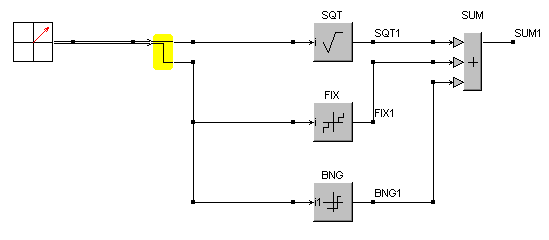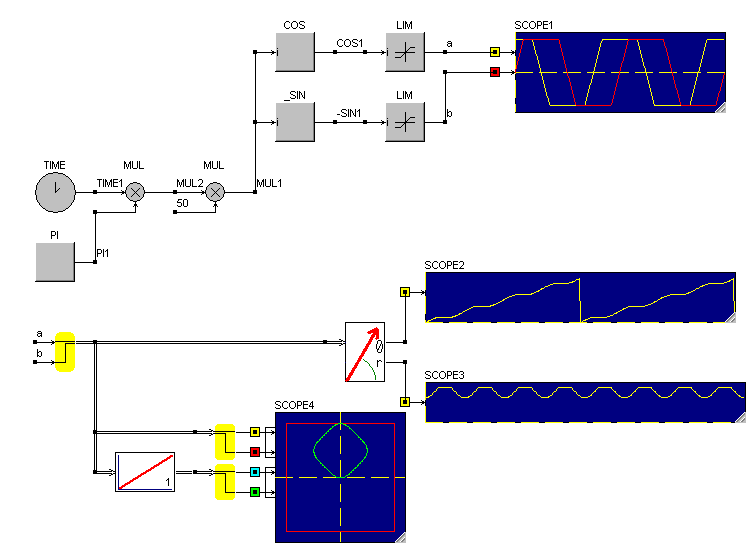• Introduction
• What is in this manual
• What is Caspoc
• User interface
• Introduction
• Starting
• Simulation
• Editing
• Viewing and printing
• Getting Started
• Basic editing
• Simulation in the time domain
• Basic User Interface Topics
• Editing
• Simulation
• Viewing
• Library
• Reports
• Project management
• Circuit and Block Diagram Components
• Introduction
• Cscript and user defined functions
• Component parameters
• Modeling Topics
• Introduction
• Power Electronics
• Semiconductors
• Electrical Machines
• Electrical drives
• Power Systems
• Mechanical Systems
• Thermal Systems
• Magnetic Circuits
• Green Energy
• Coupling to FEM
• Experimenter
• Analog hardware description language
• Embedded C code Export
• Coupling to Spice
• Small Signal Analysis
• Matlab coupling
• Tips and tricks
• Appendices

## Mixing the space vector format with the block diagram.

There are many blocks available in the standard block-diagram library. You can mix these blocks with the components from the Advanced Electrical Drives library. The difference between the space vector components and the scalar block-diagram components is that the former requires two signals to be passed from component to component.
Therefore the space vector components have multiplexed input and outputs that you can recognize by the double lines in the connections. The scalar components and the components from the block diagram have a single line. The interface between the scalar and space vector format is given by the blocks Vector2Scalar and Scalar2Vector from the section "Components/Library/AdvancedElectricalDrives/Interface".

The simulation below shows how block diagram blocks are connected to the space vector output via the Vector2Scalar component.Signals constructed in the block diagram can be passed to the space vector components using the Scalar2Vector component. In the simulation below a non-linear signal (a,b) is build and this signal is analyzed using components from the Advanced Electrical Drives library.